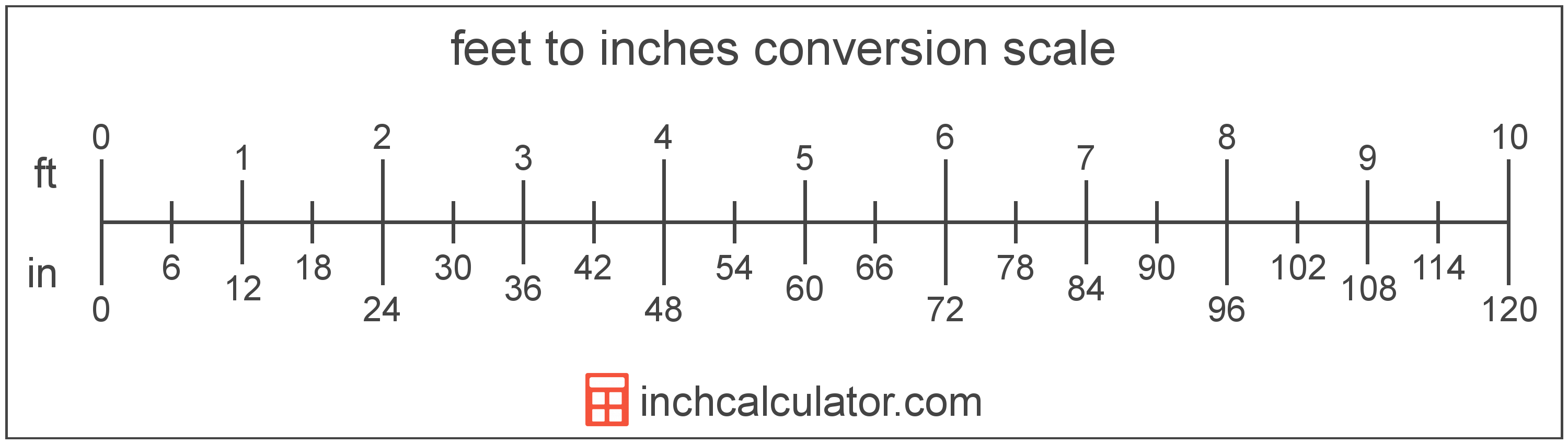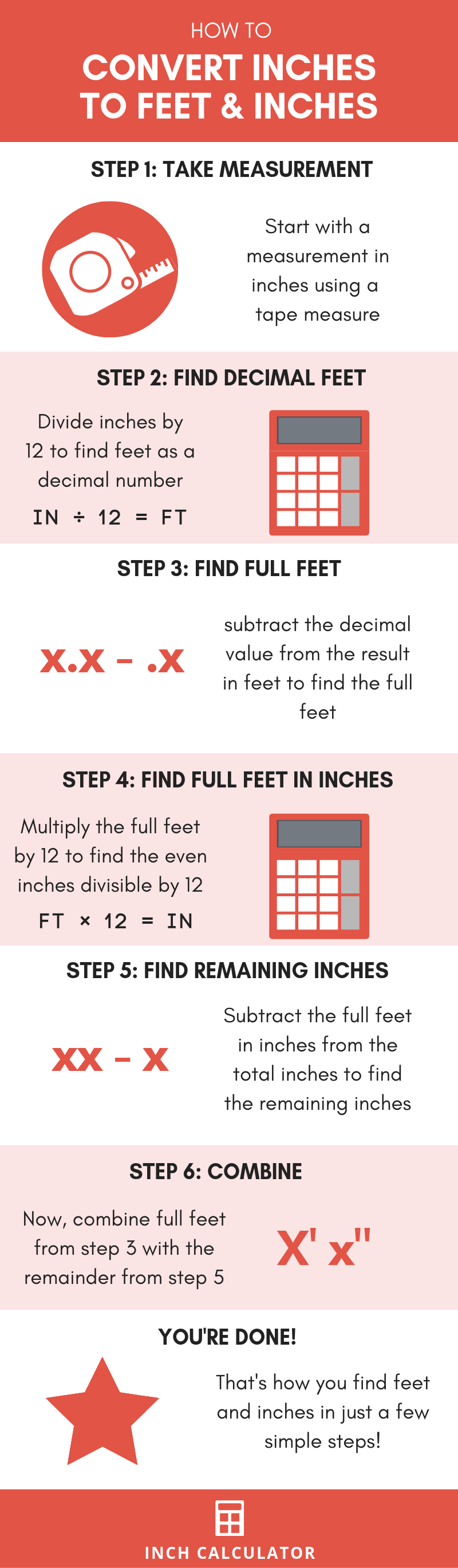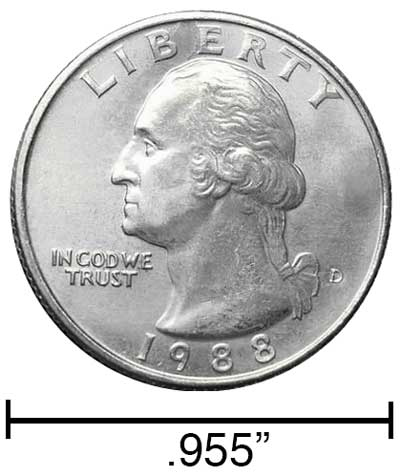# Inches to Feet Conversion Calculator

Enter the length in inches below to get the value converted to feet.

/
Results in Feet:18 in = 1.5 ft
18 in = 1' 6"
Do you want to convert feet to inches?

## How to Convert Inches to FeetConvert inches to feet and inches with this simple formula:

feet = inches ÷ 12

Converting an inch length measurement to a feet is as simple as dividing the number of inches by 12, because there are 12 inches in a foot.

For example, here's how to convert 32 inches to feet using this method.
feet = 32" ÷ 12
feet = 2.67', or 2' 8"

### How to Convert to a Mixed Measurement

Usually a decimal foot measurement is expressed as a measurement in feet and inches. For example, 2.25 ft. is often expressed as 2' 3". Follow these steps to convert inches to feet and inches.

• Divide the total inches by 12 to find the feet in a decimal form
32" ÷ 12 = 2.67'
• If the feet measurement does not have a decimal, then you're done!
• If the feet measurement does have a decimal, then subtract the decimal portion from the feet measurement to get an even feet measurement.
2.67' - .67' = 2'
• Multiply the even feet measurement by 12 to find how many of the total inches are divisible by 12.
2' × 12 = 24"
• Subtract the number of inches in the even foot measurement from the total inches to find the remainder. This is the inch measurement.
32" - 24" = 8"
• Now, take the even feet measurement and the remainder in inches. This is the feet and inches measurement.
32" = 2' 8"
• A far simpler way to get a mixed measurement is to use long division. The resulting quotient is the whole feet and the remainder is the inches.

Our inch fraction calculator can add inches and feet together and it also automatically converts the results to US customary, imperial and SI metric values.

## How do you Convert Feet and Inches to Feet?

To convert feet and inches to a decimal foot value, follow a few easy steps.

Step One - multiply the foot value by 12 to convert the value to inches.

Step Two - add the feet converted to inches with the other inch value.

Step Three - divide the total inches by 12 to find the resulting value in feet as a decimal.

For example, here's how to convert 2' 8" to feet using this method.

First, find the total inches.

in = (2' × 12) + 8"
in = 24" + 8"
in = 32"

Then, convert the total inches to feet.

ft = 32" ÷ 12
in = 2.67'## Inches

An inch is a unit of linear length measure equal to 1/12 of a foot or 1/36 of a yard. Because the international yard is legally defined to be equal to exactly 0.9144 meters, one inch is equal to 2.54 centimeters.

The inch is a US customary and imperial unit of length. Inches can be abbreviated as in; for example, 1 inch can be written as 1 in.

Inches can also be denoted using the symbol, otherwise known as a double-prime. Often a double-quote (") is used instead of a double-prime for convenience. A double-prime is commonly used to express 1 in as 1″.The standard ruler has 12", and is a common measuring tool for measuring inches. They are also often measured using tape measures, which commonly come in lengths from 6' - 35'. Other types of measuring devices include scales, calipers, measuring wheels, micrometers, yardsticks, and even lasers.

## Feet

The foot is a unit of linear length measure equal to 12 inches or 1/3 of a yard. Because the international yard is legally defined to be equal to exactly 0.9144 meters, one foot is equal to 0.3048 meters.

The foot is a US customary and imperial unit of length. Feet can be abbreviated as ft; for example, 1 foot can be written as 1 ft.

Feet can also be denoted using the symbol, otherwise known as a prime, though a single-quote (') is often used instead of the prime symbol for convenience. Using the prime symbol, 1 ft can be written as 1′.

The foot is most commonly measured using either a standard 12" ruler or a tape measure, though there are many other measuring devices available. Feet are sometimes referred to as linear feet, which are simply the measurement of length in feet.

You might be interested in our feet and inches calculator, which can add feet with inches, centimeters, or meters.

We recommend using a ruler or tape measure for measuring length, which can be found at a local retailer or home center. Rulers are available in imperial, metric, or combination with both values, so make sure you get the correct type for your needs.

Need a ruler? Try our free downloadable and printable rulers, which include both imperial and metric measurements.

## Inch to Foot Conversion Table

Inch measurements converted to feet
Inches Feet
12" 1'
24" 2'
36" 3'
48" 4'
60" 5'
72" 6'
84" 7'
96" 8'
108" 9'
120" 10'
132" 11'
144" 12'
156" 13'
168" 14'
180" 15'
192" 16'
204" 17'
216" 18'
228" 19'
240" 20'

## References

1. National Institute of Standards and Technology, Checking the Net Contents of Packaged Goods, Handbook 133 - 2019 Edition, https://nvlpubs.nist.gov/nistpubs/hb/2019/NIST.HB.133-2019.pdf
2. National Institute of Standards and Technology, U.S. Survey Foot: Revised Unit Conversion Factors, https://www.nist.gov/pml/us-surveyfoot/revised-unit-conversion-factors